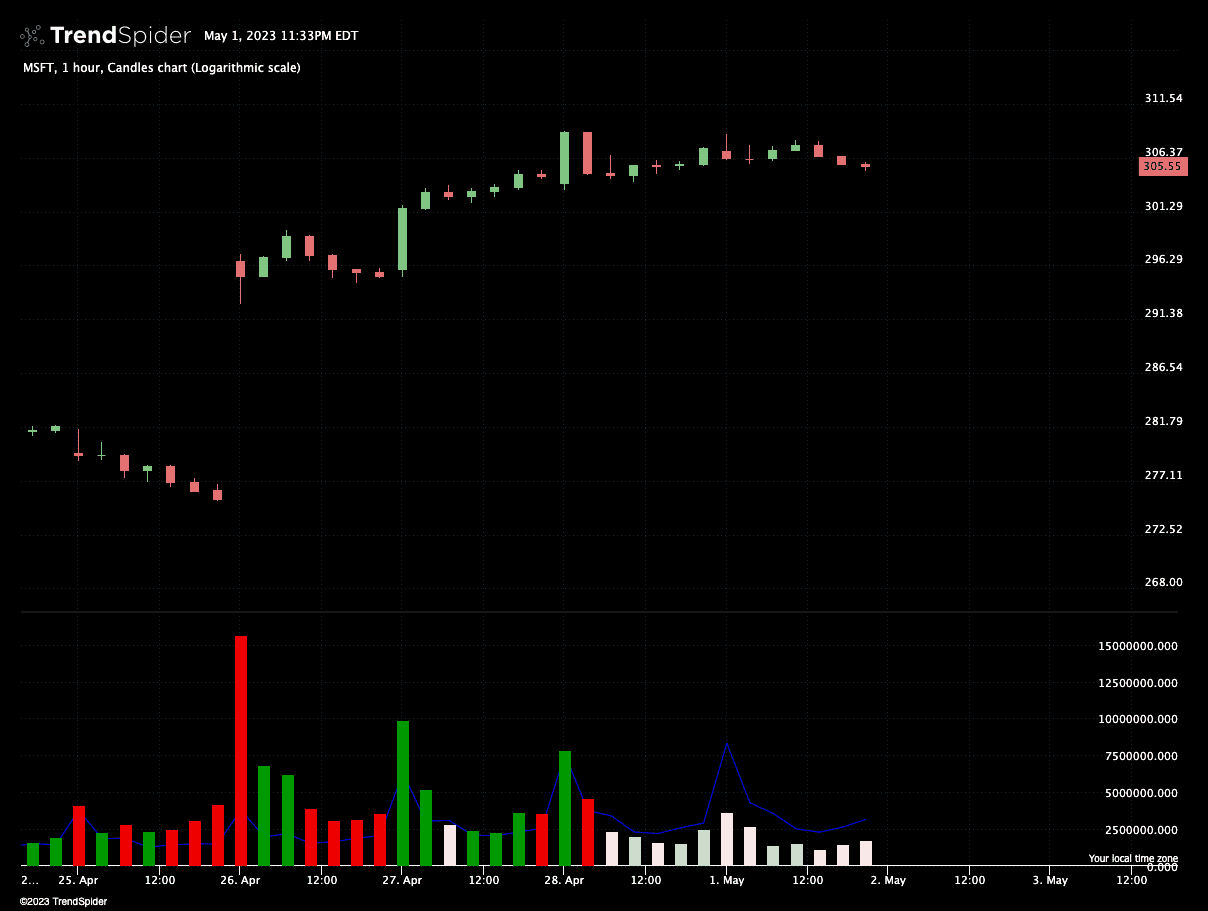# Typical Volume-by-Time Comparison Indicator

This is a volume indicator that demonstrates how to compare against the past. It compares the current intraday period's volume against the same period's volume on 5 previous trading days, and generates a visually interesting volume bar graph to illustrate the data.``````//	This indicator paints Volume histogram with an "average volume for given time" line atop of it.
//	This average volume is average volume for a given timeof a day (ie, 09:30) at a given time frame.
//	In caseif the crrent candle's volume is aboe the "typical volume for that time of a day" then its
//	histogram column will be dark. Otherwise, it will be pale.
//
//	Example of reading it: avg volume for 09:30 at SPY,10 is 2.45M. Today it's 2.0M which is below the
//	average, so the voluem column will be pale.

describe_indicator('Volume by time', 'lower');

if (isNaN(constants.resolution)) {
throw Error('This indicator can only work on intraday charts');
}

const tinycolor = library('tinycolor2');

const volumeByTime = {};
const lookbackPeriod = input('Lookback', 5, { min: 1, max: 10 });

const averageVolumes = for_every(volume, time, (v, t) => {
const timeOfDay = time_of(t);
const timeHash = `\${timeOfDay.hours}:\${timeOfDay.minutes}`;

if (!volumeByTime[timeHash]) {
volumeByTime[timeHash] = [];
}

const avgVolume = volumeByTime[timeHash].
slice(-lookbackPeriod).
reduce((result, value) => result + value, 0) / lookbackPeriod;

volumeByTime[timeHash].push(v);

return volumeByTime[timeHash].length >= lookbackPeriod + 1
? avgVolume
: null;
});

const volumeColors = for_every(close, open, averageVolumes, volume, (c, o, avg, vol) => {
if (vol > avg) {
return c > o ? '#009900' : '#ee0000';
}

return c > o ? '#cddfcc' : '#ffe7e7';
});

paint(averageVolumes, { style: 'line', color: 'blue' });
paint(volume, { style: 'histogram', color: volumeColors });``````